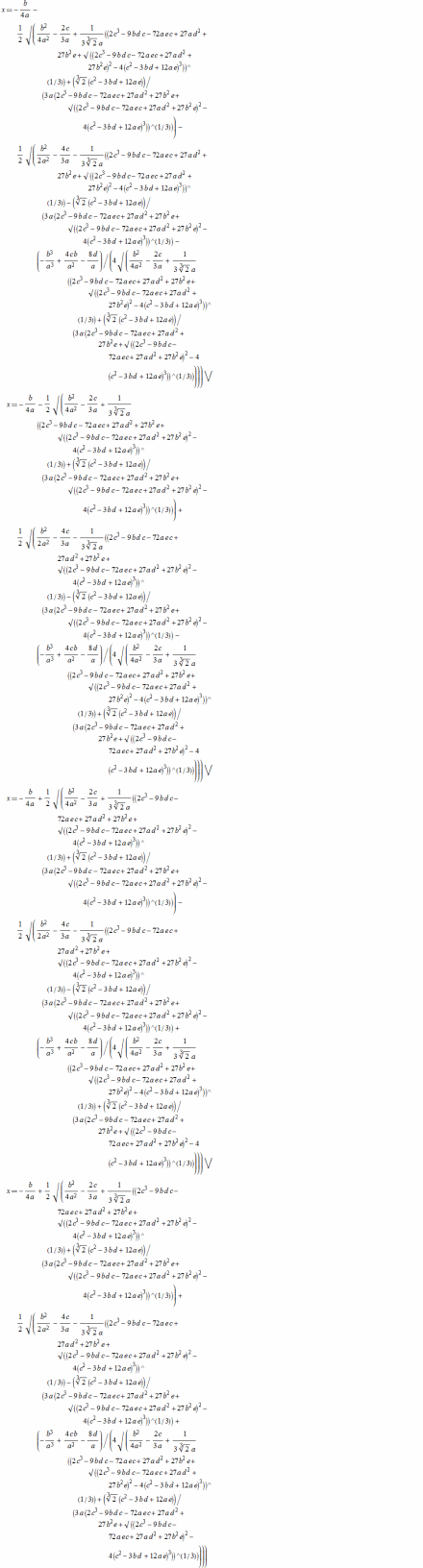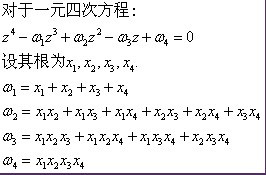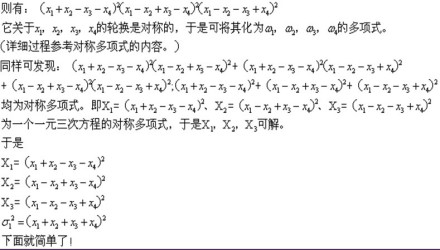• 一元二次方程 小结 一、一元二次方程 含义及特点 ...二、一元二次方程求根公式小结 叮嘟！这里是小啊呜的学习课程资料整理。好记性不如烂笔头，今天也是努力进步的一天。一起加油进阶吧！


一元二次方程的解 小结
一、一元二次方程的解 含义及特点判别式韦达定理
二、一元二次方程求根公式小结

叮嘟！这里是小啊呜的学习课程资料整理。好记性不如烂笔头，今天也是努力进步的一天。一起加油进阶吧！一、一元二次方程的解 含义及特点
（1）一元二次方程的解（根）的意义：能使一元二次方程左右两边相等的未知数的值称为一元二次方程的解。一般情况下，一元二次方程的解也称为一元二次方程的根（只含有一个未知数的方程的解也叫做这个方程的根） 。
（2）由代数基本定理，一元二次方程有且仅有两个根（重根按重数计算），根的情况由判别式决定 。判别式
利用一元二次方程根的判别式（ ）可以判断方程的根的情况  。 一元二次方程 的根与根的判别式 有如下关系：
①当 判别式 > 0时，方程有两个不相等的实数根；
②当 判别式 = 0 时，方程有两个相等的实数根；
③当 判别式 < 0时，方程无实数根，但有2个共轭复根。
上述结论反过来也成立。
韦达定理二、一元二次方程求根公式小结Ending！ 更多课程知识学习记录随后再来吧！
就酱，嘎啦！注： 1、人生在勤，不索何获。 2、一元二次方程详细参见百度百科：
https://baike.baidu.com/item/%E4%B8%80%E5%85%83%E4%BA%8C%E6%AC%A1%E6%96%B9%E7%A8%8B/7231190?fr=aladdin

展开全文数学
•文档
• 利用C语言判断一元二次方程的情况，并且一元二次方程
• 要得到一元方程求根公式，就得先定义什么是一元方程，什么是求根公式方程是指等式连接的两个式子（相信大家都明白），一元方程是指方程中只含有一个未知数的方程求根公式就是通过方程的系数进行有限加减乘除...
最近看了看方程的求解方法，感觉挺有意思的，加之最近新换了实习，又要写毕业论文，实在太忙，没时间写博客，就拿这个写一篇博客吧
方程的求根公式
要得到一元方程的求根公式，就得先定义什么是一元方程，什么是求根公式。方程是指等式连接的两个式子（相信大家都明白），一元方程是指方程中只含有一个未知数的方程。求根公式就是通过方程的系数进行有限次加减乘除开方运算得到的根的值的公式。重点是有限次加减乘除开方，这些运算都被定义为初等运算。
韦恩公式
韦恩公式指出，一元

n

n

次方程有

n

n

个根（有可能有重根，重根算多个），这是因为一元多次方程可以写成元和根相减相乘的形式：

(

x

−

r

1

)

(

x

−

r

2

)

⋯

(

x

−

r

n

)

=

0

(x-r_1)(x-r_2)\cdots(x-r_n)=0

展开之后可以得到根的和和根的积与系数的关系。
一元一次方程
形如

a

x

+

b

=

0

,

a

≠

0

ax+b=0,a \neq 0

的方程被称做一元一次方程，它的求根公式是

x

=

−

b

a

x=-\frac{b}{a}

一元方程的意义是通过求解一元方程，人们知道了负数和分数。
一元二次方程
形如

a

x

2

+

b

x

+

c

=

0

,

a

≠

0

ax^2+bx+c=0,a \neq 0

的方程被称做一元二次方程，求解它运用到的技巧就是配方法

x

2

+

b

2

a

+

(

b

2

a

)

2

=

(

b

2

a

)

2

−

c

a

x^2+\frac{b}{2a}+(\frac{b}{2a})^2=(\frac{b}{2a})^2-\frac{c}{a}

(

x

+

b

2

a

)

2

=

b

2

−

4

a

c

(

2

a

)

2

(x+\frac{b}{2a})^2=\frac{b^2-4ac}{(2a)^2}

x

=

−

b

±

b

2

−

4

a

c

2

a

x=\frac{-b\pm\sqrt{b^2-4ac}}{2a}

二元方程的意义是通过求解一元方程，人们知道了无理数（发现二次方程求根公式时人们还不知道有虚数，因为可以通过根的判别式

Δ

=

b

2

−

4

a

c

\Delta=b^2-4ac

是否大于0，来确定方程有没有实数根而避免得到复数根）。
一元三次方程
形如

a

x

3

+

b

x

2

+

c

x

+

d

=

0

,

a

≠

0

ax^3+bx^2+cx+d=0, a \neq 0

的方程被称做一元三次方程。一元一次二次方程求解都比较容易，三次方程求解很需要技巧了，首先我们可以通过变量代换来降低方程的复杂度，将

x

=

y

−

b

3

a

x=y-\frac{b}{3a}

来把方程的二次项消掉，然后把方程左右两边都除

a

a

使得最高次项变为1，得到

y

3

=

p

y

+

q

y^3=py+q

显然

p

p

和

q

q

都可以通过

a

,

b

,

c

a,b,c

来表示。然后到最巧妙的地方了，因为我们知道立方展开公式：

(

m

+

n

)

3

=

m

3

+

3

m

2

n

+

3

m

b

3

+

n

3

=

3

m

n

(

m

+

n

)

+

(

m

3

+

n

3

)

(m+n)^3=m^3+3m^2n+3mb^3+n^3=3mn(m+n)+(m^3+n^3)

这样，设

y

=

m

+

n

y=m+n

，那么

p

=

3

m

n

p=3mn

q

=

m

3

+

n

3

q=m^3+n^3

也就是说

m

3

n

3

=

q

3

27

m^3n^3=\frac{q^3}{27}

m

3

+

n

3

=

q

m^3+n^3=q

根据韦恩公式我们知道这就一元二次方程的两根之和以及两根之积的形式，通过一元二次方程的求根公式我们可以得到

m

3

m^3

和

n

3

n^3

。但是韦恩公式也告诉我们，一元三次方程应该有3个根，这说明

x

3

=

1

x^3=1

不只有一个根1，那缺了啥根？立方差公式我们可以得

x

3

−

1

=

(

x

−

1

)

(

x

2

+

x

+

1

)

x^3-1=(x-1)(x^2+x+1)

这说明

x

2

+

x

+

1

x^2+x+1

还隐藏了两个非实数根，我们定义虚数

i

=

−

1

i=\sqrt{-1}

，然后通过二次方程的求根公式可以得到另外两个虚数根从而求方程的三个根。 我们再理一下逻辑，首先通过变量代换将一般的三次方程变为没有没有二次项的三次方程，再通过立方展开公式将三次方程降次到二次方程从而可解，然后的到其中一个根（不妨设为

m

3

m^3

）开三次放得到三个值，然后通过

p

=

3

m

n

p=3mn

得到对应的

n

n

，然后

x

=

y

−

b

3

a

=

m

+

n

−

b

3

a

x=y-\frac{b}{3a}=m+n-\frac{b}{3a}

得到原方程的根。
一元四次方程
形如

a

x

4

+

b

x

3

+

c

x

2

+

d

x

+

e

=

0

,

a

≠

0

ax^4+bx^3+cx^2+dx+e=0, a \neq 0

的方程被称做一元四次方程。和处理三次方程一样，我们可以通过变量替换法消掉立方项从而得到

x

4

+

p

x

2

+

q

x

+

r

=

0

x^4+px^2+qx+r=0

拉普拉斯给出了一个很巧妙的解法，首先，把上面的方程变为

x

4

+

p

x

2

+

q

x

+

r

=

(

x

2

+

m

x

+

n

)

(

x

2

+

k

x

+

l

)

x^4+px^2+qx+r=(x^2+mx+n)(x^2+kx+l)

如果能找到这样的变换，二次方程我们又会解，那岂不是能得到原方程的解么。下面我们的问题就变成了求

m

,

n

,

k

,

l

m,n,k,l

和

p

,

q

,

r

p,q,r

的关系，根据对应次项的系数相等的关系可得：

{

m

+

k

=

0

l

+

n

+

m

k

=

p

m

l

+

k

n

=

q

l

n

=

r

\begin{cases} m+k=0 \\ l+n+mk=p \\ ml+kn=q \\ ln=r \end{cases}

变换可得

{

m

=

−

k

n

+

l

=

p

+

k

2

n

−

l

=

q

k

l

n

=

r

\begin{cases} m=-k \\ n+l=p+k^2 \\ n-l=\frac{q}{k} \\ ln=r \end{cases}

把他们化成只含有

k

k

的形式可得

{

m

=

−

k

n

=

1

2

(

k

2

+

p

+

q

k

)

l

=

1

2

(

k

2

+

p

−

q

k

)

(

k

2

+

p

+

q

k

)

(

k

2

+

p

−

q

k

)

=

4

r

\begin{cases} m=-k \\ n=\frac{1}{2}(k^2+p+\frac{q}{k}) \\ l=\frac{1}{2}(k^2+p-\frac{q}{k}) \\ (k^2+p+\frac{q}{k})(k^2+p-\frac{q}{k}) =4r \end{cases}

展开最后一个等式可得

k

6

+

2

p

k

4

+

(

p

2

−

4

r

)

k

2

−

q

2

k^6+2pk^4+(p^2-4r)k^2-q^2

这个对于

k

2

k^2

来说是个三次方程。我们已经知道三次方程的求根公式，继而可以得到

k

k

，得到

k

k

之后就可以得到

m

,

n

,

l

m,n,l

，从而可以把四次方程变为两个二次方程的乘积，二次方程我们又可解，从而能得到四次方程的求根公式。
一元五次及以上方程
一元五次方程没有一般的求根公式，但为啥没有求根公式一直困扰着人们。直到群论的出现才使得解开了这个问题的真相。群论本科时候选数学二学位学过，研究生也选了近世代数，但是不幸的是。。。都忘光了。但是大概的意思我还记得，我就简要说一下我的理解。根据韦恩公式我们可以知道，系数其实就是根的和与积的一些组合，但是这些关系有个特点，就是有对称性，也就是说，

x

+

y

=

a

,

x

y

=

b

x+y=a,xy=b

，把

x

,

y

x,y

的值交换并不影响等式的成立，也就是说无法通过系数直接求出每个根对应的值，如果想求出他们，就得破除这些对称性，例如得到

x

−

y

=

c

x-y=c

，这样才能得到对应的值，而五次方程及以没办法构造这种不对称性，所以没有一般的求根公式。
求根公式的意义
人们通过推导求根公式，不断扩充了数域，自然数（不算是数域，因为对于加减乘除不封闭）–>整数–>有理数域（一元一次方程）–>实数域（一元二次方程）–>复数域（一元三次方程），最后发展出了群论。感觉有空可以复习一下群论，这帮人真是太聪明了。
展开全文求解
• 一元二次方程ax^2+bx+c=0中, 一元二次方程求根公式： 两x1,x2= [-b±√(b^2-4ac)]/2a 韦达定理： 两x1,x2有如下关系: x1+x2=-b/a,x1*x2=c/a
一元二次方程ax^2+bx+c=0中,

一元二次方程求根公式：

两根x1,x2= [-b±√(b^2-4ac)]/2a

韦达定理：

两根x1,x2有如下关系:

x1+x2=-b/a,x1*x2=c/a
展开全文• 一元四次方程是未知数最高次数不超过四次的多项式方程，应用化四次为二次的方法，结合盛金公式求解。适用未知数最高次项的次数不大于四的多项式方程。其解法是受一元三次方程求解方法的启发而得到的。除最初解法外，...

一元四次方程求根公式，是数学代数学基本公式，由意大利数学家费拉里首次提出证明。一元四次方程是未知数最高次数不超过四次的多项式方程，应用化四次为二次的方法，结合盛金公式求解。适用未知数最高次项的次数不大于四的多项式方程。其解法是受一元三次方程求解方法的启发而得到的。除最初解法外，该方程是还有其他简便解法。
中文名
一元四次方程求根公式
提出者
费拉里适用领域
未知数最高次数不超过四次的多项式方程
应用学科
数学代数，物理学
一元四次方程求根公式来源
编辑
语音意大利数学家费拉里与一元四次方程的解法 卡当在《重要的艺术》一书中公布了塔塔利亚发现的一元三次方程求根公式之后，塔塔利亚谴责卡当背信弃义，提出要与卡当进行辩论与比赛。这场辩论与比赛在米兰市的教堂进行，代表卡当出场的是卡当的学生费拉里。 费拉里(Ferrari L.,1522~1565)出身贫苦，少年时代曾作为卡当的仆人。卡当的数学研究引起了他对数学的热爱，当其数学才能被卡当发现后，卡当就收他作了学生。 费拉里代替卡当与塔塔利亚辩论并比赛时，风华正茂，他不仅掌握了一元三次方程的解法，而且掌握了一元四次方程的解法，因而在辩论与比赛中取得了胜利，并由此当上了波伦亚大学的数学教授。 一元四次方程的求解方法，是受一元三次方程求解方法的启发而得到的。一元三次方程是在进行了巧妙的换元之后，把问题归结成了一元二次方程从而得解的。于是，如果能够巧妙地把一元四次方程转化为一元三次方程或一元二次方程，就可以利用已知的公式求解了。
一元四次方程求根公式费拉里法
编辑
语音
费拉里的方法是这样的：方程两边同时除以最高次项的系数可得 x^4+bx^3+cx^2+dx+e=0 (1)移项可得 x^4+bx^3=-cx^2-dx-e (2) 两边同时加上(1/2bx)^2 ，可将(2)式左边配成完全平方，方程成为 (x^2+1/2bx)^2=(1/4b^2-c)x^2-dx-e (3) 在(3)式两边同时加上(x^2+1/2bx)y+1/4y^2 可得 [(x^2+1/2bx)+1/2y]^2= (1/4b^2-c+y)x^2+(1/2by-d)x+1/4y^2-e (4) (4)式中的y是一个参数。当(4)式中的x为原方程的根时，不论y取什么值，(4)式都应成立。特别，如果所取的y值使(4)式右边关于x的二次三项式也能变成一个完全平方式，则对(4)对两边同时开方可以得到次数较低的方程。 为了使(4)式右边关于x的二次三项式也能变成一个完全平方式，只需使它的判别式变成0，即 (1/2by-d)^2-4(1/4b^2-c+y)(1/4y^2-e)=0 (5) 这是关于y的一元三次方程，可以通过塔塔利亚公式来求出y应取的实数值。 把由(5)式求出的y值代入(4)式后，(4)式的两边都成为完全平方，两边开方，可以得到两个关于x的一元二次方程。解这两个一元二次方程，就可以得出原方程的四个根。 费拉里发现的上述解法的创造性及巧妙之处在于：第一次配方得到(3)式后引进参数y，并再次配方把(3)式的左边配成含有参数y的完全平方，即得到(4)式，再利用(5)式使(4)的右边也成为完全平方，从而把一个一元四次方程的求解问题化成了一个一元三次方程及两个一元二次方程的求解问题。
误用：
不幸的是，就象塔塔利亚发现的一元三次方程求根公式被误称为卡当公式一样，费拉里发现的一元四次方程求解方法也曾被误认为是波培拉发现的。
一元四次方程求根公式置换群法
编辑
语音
解法见图片说明：X1，X2，X3是某个三次方程的对称多项式(X1+X2+X3，X1*X2+X2*X3+X3*X1，X1*X2*X3均可求)，利用三次方程求根公式解出X1,X2,X3；又有X=x1+x2+x3+x4=ω1，接下来根据X,X1,X2,X3解x1,x2,x3,x4一元四次方程求根公式天珩公式
编辑
语音
受费拉里法等一元四次方程求根公式的启发，沈天珩对公式进行了简化，并给出了更方便判断方程实数解个数和重根情况的判别法则。且天珩公式中不存在任何虚数开方的情况，使运算更为简单方便，也能借此简化计算机求根工具的代码。以下是完整公式：
一般式：重根判别式：总判别式：(1)当D=E=F=0时，方程有一个四重实根。(2)当DEF≠0，A=B=C=0时，方程有四个实根，其中有一个三重根。(3)当E=F=0，D≠0时，方程有两对二重根；若D>0，根为实数；若D<0，根为虚数。(4)当ABC≠0，Δ=0时，方程有一对二重实根；若AB>0，则其余两根为不等实根；若AB<0，则其余两根为共轭虚根。(5)当Δ>0时，方程有两个不等实根和一对共轭虚根。
令则有：其中，sgn表示符号因子。计算方法如下：
<1>当n=0时，sgn(n)=0。
<2>当n≠0时，sgn(n)=abs(n)/n，即 (下同)
(6)当Δ<0时，若D与F均为正数，则方程有四个不等实根；否则方程有两对不等共轭虚根。
令<1>若E=0,D>0,F>0，<2>若E=0,D<0,F>0，<3>若E=0,F<0，<4>若E≠0，一定存在；故若D或F中有非正值即方程无实数解时，，而始终为正。
此时有：
当D与F均为正时，四实根为：当D或F中有非正值时，四虚根为：公式中的总判别式与三次方程盛金公式中的以及二次方程求根公式中的极为相似，体现了数学中的有序、对称、和谐与简洁美。
一元四次方程求根公式费拉里法求根公式
编辑
语音


四次方程的求根公式过于复杂。为了描述方便，不得不借助几个中间变量。或(取模较大的数值)(若 u 为零，则 v 也取值为零)上面三个公式中，k 可取值 1,2,3。(m,S,T)的取值最好选择最大的一组，这样计算 T 时数值最稳定。如果三个均为零，则上面三个变量按下面三个公式取值四个根为(下式中)一元四次方程求根公式其它证明方法
编辑
语音
维基百科上有一个非常复杂的公式。
它是方程的四个根。将其拆分后，可得：四个根为(下式中)可见，这个公式是“求根公式(费拉里法)”的一个特例。这个公式不仅复杂、难用，而且还不能处理 m = 0 的情况，如：求解方程(等价于方程)时会失败。
词条图册
更多图册
参考资料
1.
中华人民共和国教育部．数学书：未知，2003

展开全文• 求根公式解一元二次方程 import math def quadratic(a, b, c): if a == 0: if b == 0: if c == 0: print('方程有任意') else: print('方程') else: x = -c / b print('方程：x=%.2f'python
• ## 解方程之求根公式

万次阅读 2020-10-04 15:06:38
一元二次ax^2 +bx+c=0可用求根公式x= 求解，它是由方程系数直接把表示出来的公式。这个公式早在公元9世纪由中亚细亚的阿尔·花拉子模给出。 拓展资料： 南宋数学家秦九韶至晚在1247 年就已经发现一元三次方程...
• 两边同时除以二次项系数 常数项移动到等号另一边 为了配方，两边都加上一次项系数的二分之一的平方 最后开方整理记得到求根公式
• ax2+bx+c=0方程，a,b,c由键盘输入，设b2-4ac>0。 求根公式为： 则x1=p+q x2=p-q 源程序如下： #include<math.h> main() { float a,b,c,disc,x1,x2,p,q; scanf(“a=%f,b=%f,c=%f”,&a,&...
• 标准型的一元次方程aX^3+bX^2+... 两种公式法都可以标准型的一元次方程。用卡尔丹公式解题方便，相比之下，盛金公式虽然形式简单，但是整体较为冗长，不方便记忆，但是实际解题更为直观。 一、卡丹公式法的...
• 简单C语言小程序：求根公式求一元二次方程式的！ 一、算法的构思 一元二次方程的一般式：ax^2+bx+c = 0 我们知道，一元二次方程）的充要条件是：b^2-4ac>=0。如果不满足此关系式，那么方程。接着当...c语言
• 一元三次方程求根公式的解法-------摘自高中数学...一元三次方程的求解公式的解法只能用归纳思维得到，即根据一元一次方程一元二次方程及特殊的高次方程求根公式的形式归纳出一元三次方程求根公式的形式。归纳出c
•算法
• 转自百度百科 公式法（卡尔丹公式） （如右图所示） 若用A、B换元后，公式可简记为： ...一元次方程求根公式判别法 当△=(q/2)^2+(p/3)^3>0时，有一个实和一对个共轭虚根； 当△=(q/2)^2+(p/3...
• 一元二次方程求根计算器，可以正常显示化简后的分式、根式结果。
• 最简单的一元二次方程求根问题的C源码，小白易懂，忽略了简洁好看，突出易懂，已在Devc++和VC++成功运行。
•wordpress chrome vue 计算机视觉 nagios
• 其中，方程根的解法更是一元二次方程的重中之重，下面就给大家分析一下一元二次方程在初中学习中常见的方程的解法：求解一元二次方程求解一元二次方程方程常见的有三种方法：（1）公式法：将一元二次方程化为一般...
• 一、特征方程与特征、特征方程与特征 示例 ( 重要 )
• 对于简单的一元次方程的迭代#include #include #include #define MAXTIMES 5typedef int times;typedef double coefficient;typedef struct _properties{coefficient x; //系数times n;//次数}......